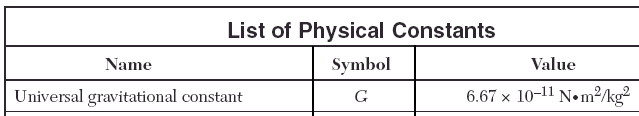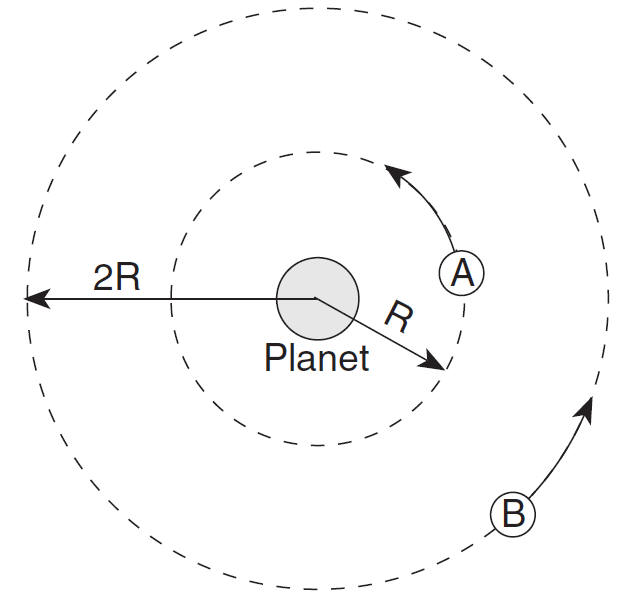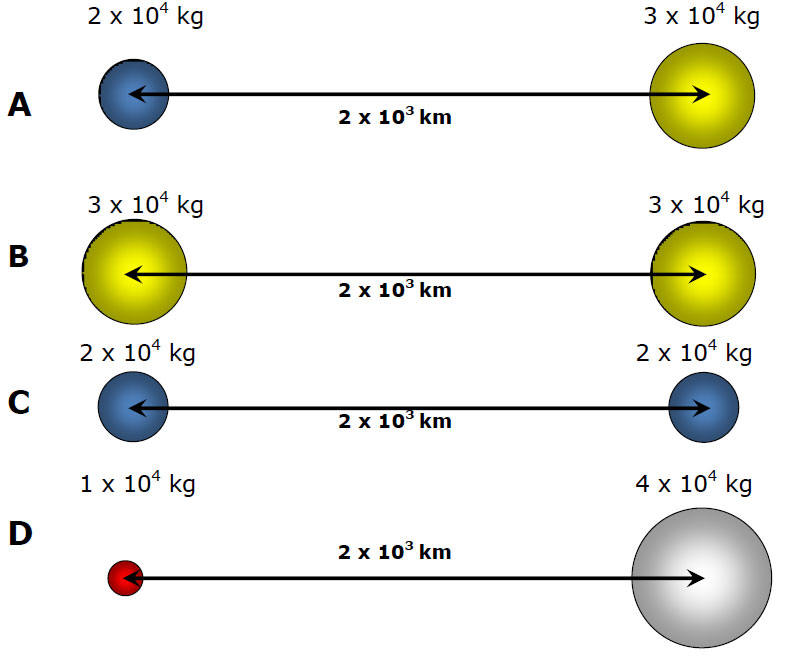HomeGravity by John Mayer IIImage found on Wikipedia

C) Universal Law of GravityGravity - mutual force of attraction between any two objects in the universe

 F = Gm1m2 (r2)

G = Gravitational constant = 6.67 x 10-11 Nm2/kg2

(Reference Table p. 1)m1, m2  two massesr - distance between
center of masses

Giancoli p. 131) 28F = Gm1m2 r2

Fg proportional to
product of two masses

 Fg m1 m2 100 N x y 200 N 2x y 600 N 2x 3y 400 N x 4y 150 N 1/2x 3yF = Gm1m2 (r2)

Fg inversely proportional to the square of distance between the object's center of massFg r x1/4 x2 x1/9 x3 x1/16 x4 x9 x1/3 x16 x1/4

Ex) If the gravitational force on satellite A is 160,000 N, what is the gravitational force on satellite B?F = 40,000 N

(twice distance, 1/4 the force)

Fluids in Micro-Gravity

Rank the gravitational forces from greatest to smallest.WeightEnrichmentwww.wpclipart.com

How do we measure very tiny gravitational forces?

Determining G, Cavendish

Dark Matter